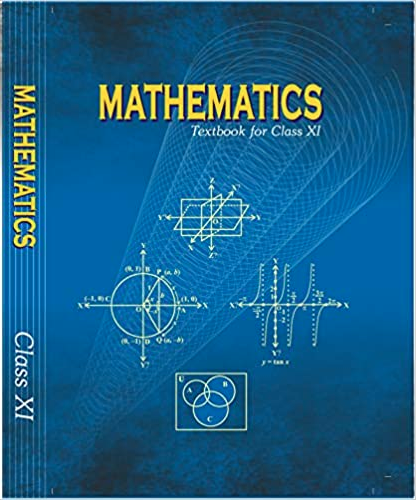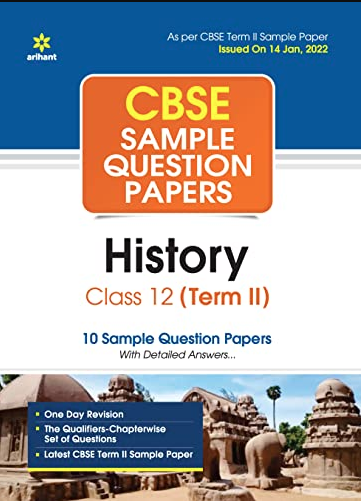# Odisha HSC /10th Class Exam Time Table 2020

Odisha HSC Exam Time Table 2020
DateDaySubject
19/02/2020WednesdayOdia Language
22/02/2020SaturdayEnglish
24/02/2020MondaySanskrit/ Hindi
26/02/2020WednesdayMathematics
28/02/2020FridayGeneral Science
03/02/2020MondaySocial Studies

Last Updated on: March 12, 2020Chapter 5 - Complex Numbers and Quadratic Equations Questions and Answers: NCERT Solutions for Class 11 MathsExercise 5.1 1. Express the given complex number in the form a+ib:(5i)(−35i)a+ib:(5i)(−35i) And evaluate Ans: Evaluate the complex number (5i)(−35i)=−5×35×i×i(5i)(−35i)=−5×35×i×i (5i)(−35i)=−3i2⋯[i2=−1](5i)(−35i)=−3i2⋯[i2=−1] (5i)(−35i)=3(5i)(−35i)=3 2. Express the given complex number in the… Read More...Chapter 4 - Principle of Mathematical Questions and Answers: NCERT Solutions for Class 11 Maths( Exercise 4.1) 1. Prove that following by using the principle of mathematical induction for all n∈Nn∈N: 1+3+32+.....+3n-1=(3n-1)21+3+32+.....+3n-1=(3n-1)2 Ans: Let us denote the given equality by P(n),P(n), i.e., P(n):1+3+32+.....+3n-1=(3n-1)2P(n):1+3+32+.....+3n-1=(3n-1)2 For… Read More...Mention any two places related to the Revolt of 1857.Mention any two places related to the Revolt of 1857. Answer. Mention any two places related to the Revolt of 1857. Jhansi, Delhi, Calcutta, Awadh, Kanpur, Meerut Read More...